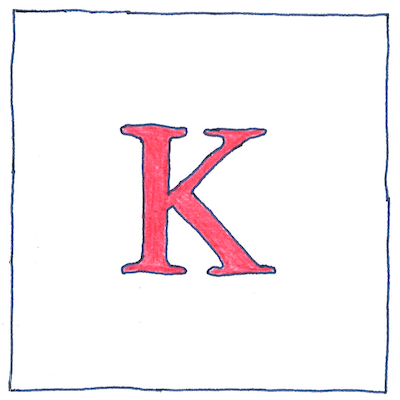# 1848

## The book of science

Tom Sharp

 William Thomson, Lord Kelvin thermometry

## Kelvin scale

• The fact that absolute zero exists
• had been established since Robert Boyle, 1665,
• although for the wrong reason. They thought then
• that all coldness was a quality communicated
• from one supremely cold body.
• Guillaume Amontons thought nothing could be below -240 °C.
• Johann Heinrich Lambert thought zero was at -270 °C.
• Pierre-Simon Laplace and Antoine Lavoisier agreed
• it must be below -600 °C but was probably
• between 1,500 to 3,000 below.
• Dalton calculated it many times
• and finally concluded it was -3000 °C.
• Kelvin calculated the value
• of absolute zero at -273 °C, and proposed
• an absolute scale starting from absolute zero
• and whose increments, anywhere in the scale,
• would produce exactly the same amount of work
• based on Sadi Carnot’s theory of the motive power of heat.

## Other definitions of absolute zero

• The temperature at which
• the volume of an ideal gas collapses to zero.
• 273.16 below the triple point of water
• at a partial vapor pressure of 611.73 pascals.
• The negative reciprocal of the expansion coefficient
• of gas for each degree Celsius relative to the ice point.
• Impossibly cold, and no colder.

## Is it not relative?

• The Celsius scale is relative to two points,
• the freezing and boiling points of water.
• Each one-hundredth of the interval between these two points
• is a Celsius degree, so the degree is relative.
• The Kelvin scale is also relative to two points,
• absolute zero and the Celsius degree.
• Without the relation to the relative degree,
• we would have no scale of kelvins.

Scientists have been able to cool copper to 0.6% of a degree Celsius from absolute zero. Ever closer, never there.

The increments of the Kelvin scale are equal to the Celsius degree. A footnote to the Kelvin scale is the Rankin scale, proposed by William John Macquorn Rankine in 1859. It is an absolute scale like the Kelvin scale, but whose increments are equal to the Fahrenheit degree.